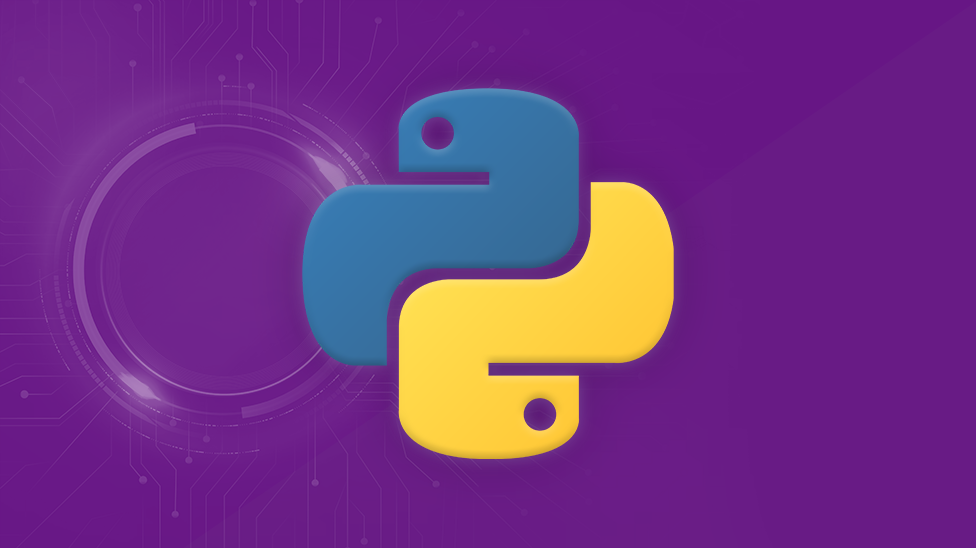# Introduction to Quantum Circuit simulation in Python - part 1

3
min de leitura

Despite the growing list of QC simulators readily available, one of the best ways to learn something is to do it yourself. In this series of posts we are going to learn exactly how to do that. We are not going to worry about performance or ways to simulate a large number of qubits, instead we will focus on a simply way to simulate smaller systems.## Prerequisites

In order to follow this series, you will need a basic understanding of the following topics:

• Python programming, of course.
• Linear algebra in the field of complex numbers ℂ.
• The very basics of quantum mechanics and quantum computing.

This Wikipedia page does a good job of introducing you to Quantum Computing. After reading that, check this to learn more about the qubit. The particularities of linear algebra in the field of complex numbers will be handled by numpy.

## The plan

After getting familiar with the topics above, we will start by implementing a Gate class, to represent our unitary operators, ie, our quantum gates. Then, we will create a Linalg class to encapsulate some linear algebra operations, like the tensor product. After that, we can adopt some data format (json for example) and apply some structure to it, in order to represent our circuit. This structure will then be parsed with the help of the two classes above. At this point, our simulator is ready to go!

## Up next

In the next part of this series we will define our circuit structure and make use of the two classes above.

See you next!

Compartilhar esse conteúdo: